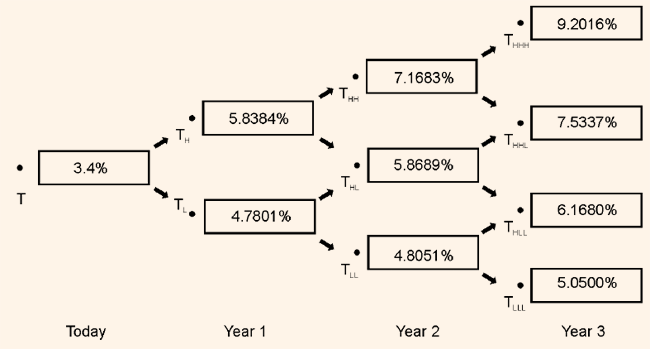## Constructing the binomial interest rate tree, Financial Management

Assignment Help:

The fundamental principle is that when a tree is used to value an on-the-run issue, the resulting value should be arbitrage free i.e., it should be equal to the observed market value. Also, the interest rate tree should be consistent with the assumed interest rate volatility.

Let us, with help of an example, look at the process of constructing an interest rate tree:

The interest rate at the first node T would be the current 1-year on-the-run issue rate. The interest rate for year-one would be calculated using the coupon rate for the 2-year on-the-run issue, assumed interest rate volatility, and the interest rate at the base of the tree. Given these, the interest rates are determined on a trial and error basis. First, the lower rate r1,L at the node TL is assume and then using the formula (discussed earlier in this chapter) the interest rate at the higher value is calculated. It is then compared with the 2-year on-the-run issue to see if there are discrepancies in both the values; it implies that the assumption made is incorrect. If the value is too high, a higher rate guess should be made and if the value is too low a lower rate guess is to be made until the value of r1,L is in line with the 2-year on-the-run issue.

In similar manner, rates are determined for year-two - r2,LL, r2,HL and r 2,HH. The information required for this task includes:

1. The coupon rate for the 3-year on-the-run issue.

2. Assumed interest rate volatility.

3. The interest rate at the base of the tree.

4. The two 1-year rates (r1,L and r1,H).

A guess is made of the value r2,LL, and based on the formula discussed earlier in this chapter, the

value of r2,HL and r 2,HH are calculated. If the value generated by this process is not equal to the market value of the 3-year on-the-run issue, the process is to be repeated again. An iterative process is again followed. Table 2 shows the binomial interest rate tree for the issuer for valuing issues up to four years of maturity assumption volatility for the 1-year rate of 10% and Table 2 verifies that the rates on the binomial interest rate tree are the correct values. This is arrived at by showing that when the 3-year on-the-run issue is valued using backward induction method the value is 100, which is nothing but the market value of the 3-year on-the-run issue.

Table 2: Binomial Interest Rate TreeAssumed Volatility = 10%

Table 2: Verification of the Rates on the Binomial Interest Rate TreeAssumed Volatility = 10%

Coupon tate = 5%

#### Two-for-one stock split, The equity accounts for Hexagon International are ...

The equity accounts for Hexagon International are as follows: a.    If Hexagon stock currently sells for \$50 per share and a 20% stock dividend is declared, how many new s

#### Bond derivatives-callable bonds , Callable bonds give the right...

Callable bonds give the right to the issuer to redeem the bond prior to its maturity date, at a specified call price. These bonds are beneficial to the

#### Budgeting, Budgeting: All business owners should recognise and understa...

Budgeting: All business owners should recognise and understand the importance of preparing and maintaining a financial budget for their business. Budgets are an essential fi

#### Advantages to a company from having a robust health, Z works for HS Company...

Z works for HS Company and has been asked to undertake an assessment of any health and safety issues that might be potential hazards in the department which she manages. Z's respon

#### Role of Trustee in Pension Fund, Role of Trustee in Pension Fund: Trust...

Role of Trustee in Pension Fund: Trustees are people in control of long-term asset allocation of a pension scheme. Whatever benchmark they set will, as we shall see, influence

#### Determine the method of credit rating, Determine the method of Credit Ratin...

Determine the method of Credit Rating It is obligatory for the issuing companies to get credit rating done on debt securities issues. Credit ratings are also required for Comme

#### Bond market innovations, As liberalization is gathering momentum, cor...

As liberalization is gathering momentum, corporate treasures and merchant bankers are in the process of devising new products to suit the needs of investors and c

#### Role of government notes and bonds in finance national debt, Explain the vi...

Explain the vital role of government notes and bonds in the finance national debt. Government notes and bonds are issued within the USA by the US Treasury to finance national d

#### Calculate the expected return and standard deviation, Benjamin Tang current...

Benjamin Tang currently has holdings in the following three companies:                                                                             E(R)                      σ

#### Explain pro forma financial statements and a cash budget, What is the diffe...

What is the difference between pro forma financial statements and a cash budget?  Explain why pro forma financial statements are not used to forecast cash needs. Pro forma